Get inspired by the success stories of our students in IIT JAM MS, ISI  MStat, CMI MSc DS.  Learn More

# ISI MStat PSB 2013 Problem 2 | Application of sandwich Theorem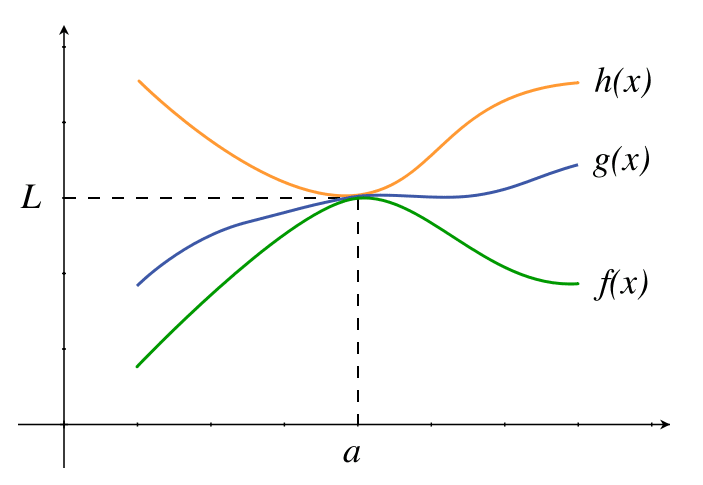This is a very beautiful sample problem from ISI MStat PSB 2013 Problem 2 based on use of Sandwich Theorem . Let's give it a try !!

## Problem- ISI MStat PSB 2013 Problem 2

Let f be a real valued function satisfying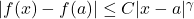for someand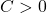(a) If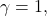show that f is continuous at a
(b) If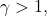show that f is differentiable at a

### Prerequisites

Differentiability

Continuity

Limit

Sandwich Theorem

## Solution :

(a) We are given that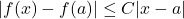for some.

We have to show that f is continuous at x=a . For this it's enough to show that.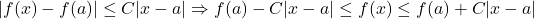Now taking limitwe have ,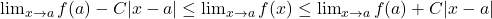Using Sandwich theorem we can say that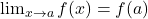. Since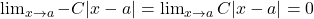Hence f is continuous at x=a proved .

(b) Here we have to show that f is differentiable at x=a for this it's enough to show that theexists .

We are given that ,for some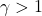and,

which implies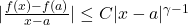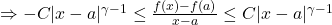Now taking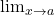we get by Sandwich theorem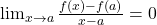i.e f'(a)=0 .

Since ,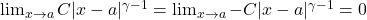, for.

Hence f is differentiable at x=a proved .

## Food For Thoughtbe such that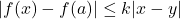for some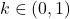and all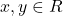. Show that f must have a unique fixed point .

## Subscribe to Cheenta at Youtube

This is a very beautiful sample problem from ISI MStat PSB 2013 Problem 2 based on use of Sandwich Theorem . Let's give it a try !!

## Problem- ISI MStat PSB 2013 Problem 2

Let f be a real valued function satisfyingfor someand(a) Ifshow that f is continuous at a
(b) Ifshow that f is differentiable at a

### Prerequisites

Differentiability

Continuity

Limit

Sandwich Theorem

## Solution :

(a) We are given thatfor some.

We have to show that f is continuous at x=a . For this it's enough to show that.Now taking limitwe have ,Using Sandwich theorem we can say that. SinceHence f is continuous at x=a proved .

(b) Here we have to show that f is differentiable at x=a for this it's enough to show that theexists .

We are given that ,for someand,

which impliesNow takingwe get by Sandwich theoremi.e f'(a)=0 .

Since ,, for.

Hence f is differentiable at x=a proved .

## Food For Thoughtbe such thatfor someand all. Show that f must have a unique fixed point .

## Subscribe to Cheenta at Youtube

This site uses Akismet to reduce spam. Learn how your comment data is processed.

### Knowledge Partner